# How do you find the derivative of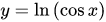?Mercedes

Solution:

Concept:The derivative of a function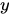is the rate of change of the function or in other words, it is the amount of change in function at a given point. It is denoted by.

The derivative of some functions are given in the table below: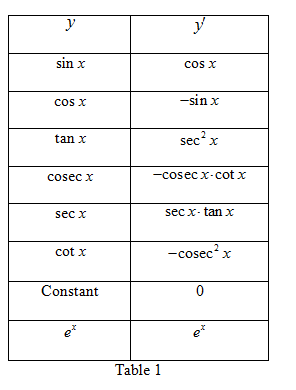Calculation:

Step:1 The function is.

Now take the derivative of the above equation.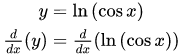Step:2 Simplify further to solve for the derivate as shown below: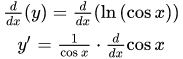Step:3 From the Table 1, the derivative of cosxis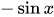. Use the value of derivative of cosx and solve further as below:The derivative valueis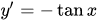.

Answer:The derivative ofis.

Similar Problems:

Problem 1:What is the derivative of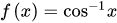?

Solution:

Step:1 The functioncan be written as given below: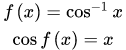Step:2 Now take the derivative of the above equation.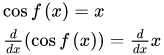Step:3 From the above Table 1 shows that the derivative of cosxis, therefore solve the derivative further as below:Since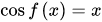, then the derivative value becomes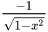.

Answer:The derivative of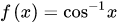is.

Problem 2:What is the derivative of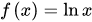?

Solution:

Step:1 The functioncan be written as given below:Step:2 Now take the derivative of the above equation.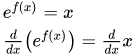Step:3 From the above Table 1 shows that the derivative of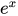is, therefore solve the derivative further as below:Since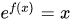, then the derivative value becomes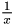.

Answer: The derivative ofis.

### Need homework help now?

tutors online

Related Questions
##### (t) in °C of the pot of soup at time t (in The initial temperature of a pot of soup is 80°C. The temperature minutes) satisfies the differential equation dT di T-20 10 (a) Solve the differential equation, and find an explicit expression for the function (). By separating variables, integrating and manipulating arbitrary constants, . We are also told that so that and so . ...
Notes
Me

OR

Don't have an account?

Join OneClass

Access over 10 million pages of study
documents for 1.3 million courses.

Join to view

OR

By registering, I agree to the Terms and Privacy Policies
Just a few more details

So we can recommend you notes for your school.